### Home > CALC > Chapter 4 > Lesson 4.1.2 > Problem4-23

4-23.
1. For parts (a) and (b) below, trace f(x) on your paper. Then, using a different color pencil or highlighter, sketch the graph of y = f ′(x) for the function given. Homework Help ✎

1.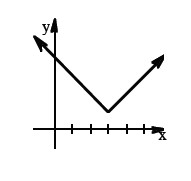2.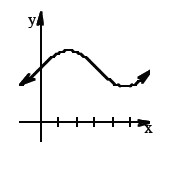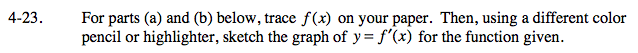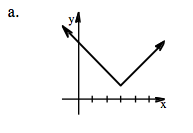f(x) has a cusp at x = 3, so x '(x) should have a jump at
x = 3.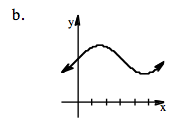The max and min on a smooth continuous graph are the location of zeros on the derivative graph.